# How to plot integration equation using Python?

• Python
I have a few of integration equations and need to convert it into Python. The problem is when I tried to plot a graph according to the equation, some of the plot is not same with the original one.

The first equation is the error probability of authentication in normal operation: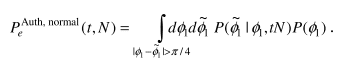cond equation is the error probability of authentication under MIM attack: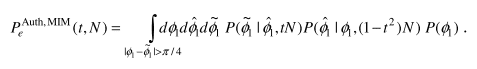The error probability of authentication in normal operation can be calculated by: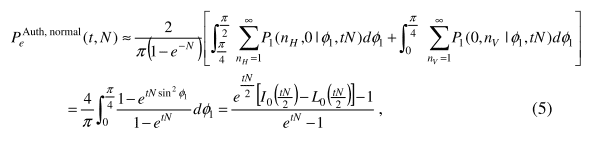Supposedly, the graph (original) will be shown like this: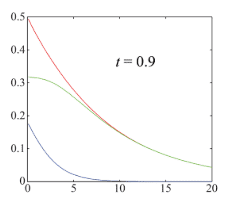y-axis: error probability
x-axis: N
Pe^normal = blue lines
Pe^MIM = red lines
Differences between two error probabilities (Pe^MIM - Pe^normal)= green lines

I tried to code it into Python and this is my full codes:

Python:
import matplotlib.pyplot as plt
import math
import numpy as np
from scipy.special import iv,modstruve

x=np.arange(-0.5,21,1)
x = np.array(x)
t = 0.9
pe_normal = (np.exp(t*x/2)*(iv(0, t*x/2) - modstruve(0,t*x/2))-1)/(np.exp(t*x)-1)
pe_diff = (np.exp((1-t**2)*x/2)*(iv(0, (1-t**2)*x/2) - modstruve(0,(1-t**2)*x/2))-1)/(np.exp((1-t**2)*x)-1)

plt.plot(x, pe_normal, '-', color='blue', label='Normal')
plt.plot(x, pe_mim,  '-', color='red', label='MIM')
plt.plot(x, pe_diff,  '-', color='green', label='DIFF')
plt.xlabel('Mean photon number N')
plt.ylabel('Error probabiity')
plt.text(10, 0.4, 't=0.9', size=12, ha='center', va='center')
plt.ylim([0, 0.5])
plt.xlim([0, 20])
plt.legend()
plt.show()

The graph produce from my code is: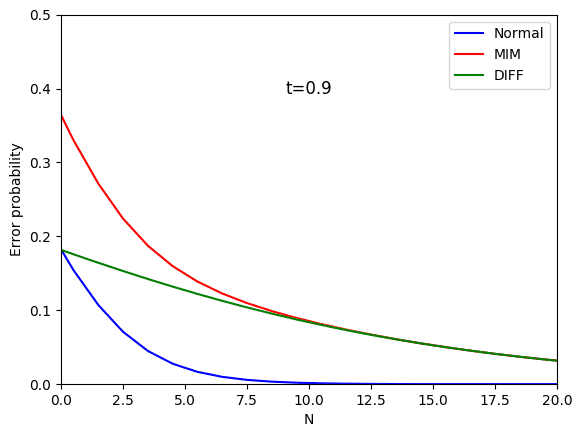It looks like that my plot is not same with the original one in terms N=0 of Pe^MIM (red line) and differences between two error probabilities (green line). The problem is the calculation for error probability under MIM attack was not given.

I hope that anyone may help me to solve this problem.

Thank you.

#### Attachments

Last edited:

Didn’t we discuss this program in this thread?

Where you never responded to say whether it helped or not or have you fixed the expression I mentioned in it?
Thank you so much for your help. Apologize for not replying the threads. This post is different from the previous one.

Mentor
Ahh okay. I’ll look at it some more. Python and it’s plot capability is really awesome.

Yes
Ahh okay. I’ll look at it some more. Python and it’s plot capability is really awesome.
Yes. It is very interesting to play around with python. However, I felt some headache to understand the equation.
Thank you for your attention and help for the previous post.
Really appreciate it.

Mentor
A couple of questions:

- so the t**2 means to square the t value right? Okay I found this is correct.

- and the z2 = y + z adds the elements of y to the elements of z to get an array of z2 right?

I thought maybe you need to use numpy to do the element by element addition.

I didn’t see the equation that you used to code the z = line

Mentor
For numpy:

Python:
z2 = np.add(y,z)

A couple of questions:

- so the t**2 means to square the t value right? Okay I found this is correct.

- and the z2 = y + z adds the elements of y to the elements of z to get an array of z2 right?

I thought maybe you need to use numpy to do the element by element addition.

I didn’t see the equation that you used to code the z = line

1. Yes. t**2 is the square the t value
2. Yes. z2 = element of y + element of z
3. z line is (1-t**2)N, which is replacing tN in Pe^(aut,normal)

Mentor
While I don’t see exactly what’s wrong, I do see places where you could improve the readability of your code.

1) use the same colors for the curves to make easier to compare the expected graph to the one you generated

2) label your variables like pe_mim, pe_normal, pe_diff

3) I don’t see where you’re doing the pe_diff calculation and this may be the source of your problem,

For numpy:

Python:
z2 = np.add(y,z)

I have tried it. However, it shows the same graph that I have been plotted before.
View attachment 236522
While I don’t see exactly what’s wrong, I do see places where you could improve the readability of your code.

1) use the same colors for the curves to make easier to compare the expected graph to the one you generated

2) label your variables like pe_mim, pe_normal, pe_diff

3) I don’t see where you’re doing the pe_diff calculation and this may be the source of your problem,

I already updated the code and the graph in the question.

2022 Award
Your curve for ##P_e^{Auth,Normal}(t,N)## looks right. Therefore the problem is in your MIM case. You don't seem to have written out an explicit expression for ##P^{Auth,MIM}_e(t,N)##. Reverse engineering your code, you have implemented $$P_e^{Auth,MIM}(t,N)= {{\left(I_0\left({{\left(1-t^2\right)N}\over{2}}\right)- L_0\left({{\left(1-t^2\right)N}\over{2}}\right) \right)e^{{{\left(1-t^2\right)N}/{2}}}-1}\over{e^{\left(1-t^ 2\right)N}-1}}+{{\left(I_0\left({{tN}\over{2}}\right)- L_0\left({{tN}\over{2}}\right)\right)e^{{{tN }/{2}}}-1}\over{e^{tN}-1}}$$(Edit: replaced x with N above.) I presume this is incorrect.

Last edited:
•jedishrfu
Your curve for ##P_e^{Auth,Normal}(t,N)## looks right. Therefore the problem is in your MIM case. You don't seem to have written out an explicit expression for ##P^{Auth,MIM}_e(t,N)##. Reverse engineering your code, it is $$P_e^{Auth,MIM}(t,N)= {{\left(I_0\left({{\left(1-t^2\right)x}\over{2}}\right)- L_0\left({{\left(1-t^2\right)x}\over{2}}\right) \right)e^{{{\left(1-t^2\right)x}/{2}}}-1}\over{e^{\left(1-t^ 2\right)x}-1}}-{{\left(I_0\left({{tx}\over{2}}\right)- L_0\left({{tx}\over{2}}\right)\right)e^{{{tx }/{2}}}-1}\over{e^{tx}-1}}$$I presume this is incorrect.

How to write the expression for ##P^{Auth,MIM}_e(t,N)##? Do you have any idea, what is the equation to plot ##P^{Auth,MIM}_e(t,N)##?

Last edited:
2022 Award
I've no idea. I simply reverse engineered your code in the hope that you would see a typo or something.

You seem to have a source with at least some of the maths in it - what does it say? And you have the graph you want to replicate - how was it generated?

2022 Award
I simply reverse engineered your code in the hope that you would see a typo or something.
...speaking of which, it should be a plus between the fractions. Now corrected above.

...speaking of which, it should be a plus between the fractions. Now corrected above.
Yes. I realized it. It is stated that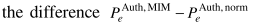. However, I don't think that they used another equation to calculate pe_mim.

#### Attachments

2022 Award
Then what is the expression given for the difference? Is it consistent with the expression I gave in #12?

Actually, I didn't have any idea.

2022 Award
Then where did you get what you implemented in the code?